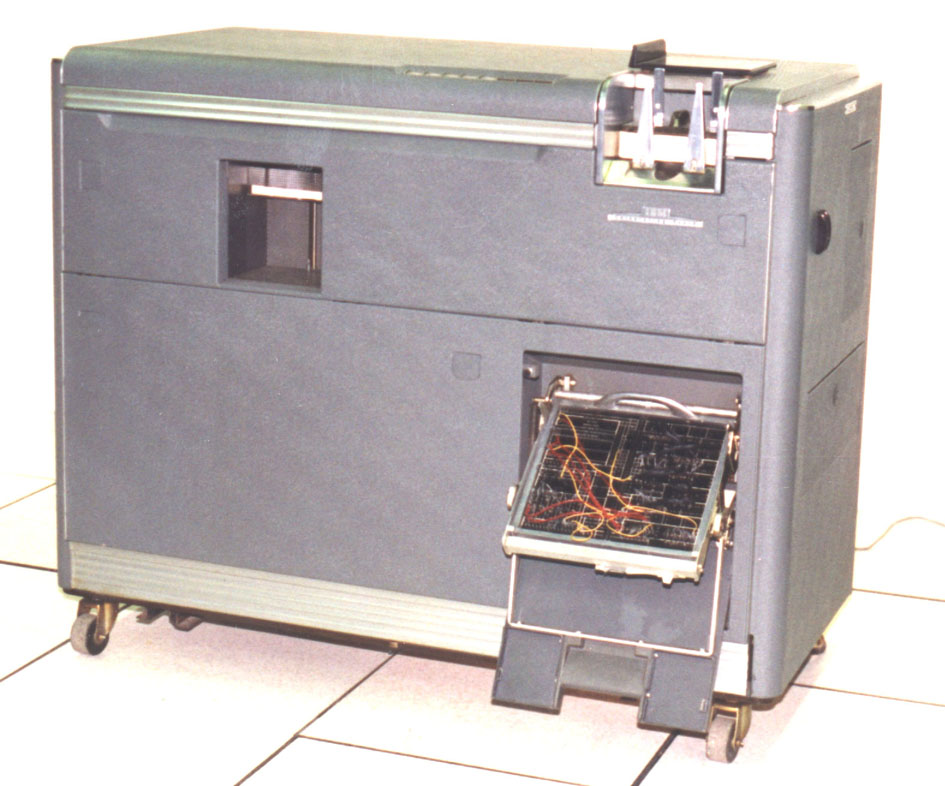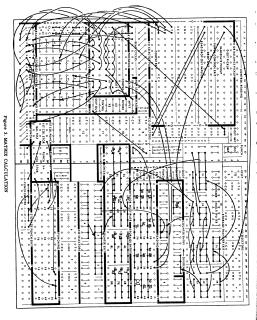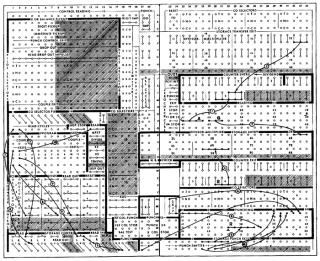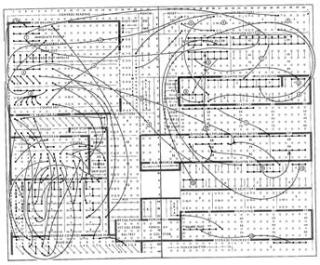YaK:: Archive of IBM 602A Calculating Punch Resources [Changes]   [Calendar]   [Search]   [Index]   [PhotoTags]

Archive of IBM 602A Calculating Punch Resources

Some is 602. Some is 602A "the 602 that works".

 PDFs: Manual for the 602: bitsavers-org-ibm602-A22-0506-1_602_Jan60.pdf Inversion of 25x25 Matrix: "About 75 calculating machine hours are required to be spent for working out the 25x25 matrix." 602a-inversion-of-matrix-1955--57.E01.pdf IBM_Computation_Seminar_Dec49.pdf IBM_Industrial_Computation_Seminar_Sep50.pdf IBM_Computation_Seminar_Aug51.pdf

•••InfoBox4: IBM602A http://www.users.nwark.com/~rcmahq/jclark/ibm602.htm : ''The IBM 602 Calculating Punch reads factors punched in IBM cards and performs mathematical operations of addition, subtraction, multiplication and division. The results of these calculations can then be punched into the same cards from which the factors were read or into trailer cards. The standard machine can multiply a 22-digit multiplican by an 8-digit multiplier to obtain a 30 digit product. A 15-digit dividend can be divided by an 8-digit divisor to obtain and punch an 8-digit quotient. All operations of the 602 are performed in steps or operating cycles which occur at the rate of 200 per minute or 12,000 per hour. The number of cycles required for a card depends on the size of the factors and the type of problem. A glance at the picture will show the card path through the 602 is not straight, but the card actually makes a right angle turn. The operations of the 602 are controlled by wiring the control panel which is inserted into the front of the machine. Below is the wiring diagram to do square root.''